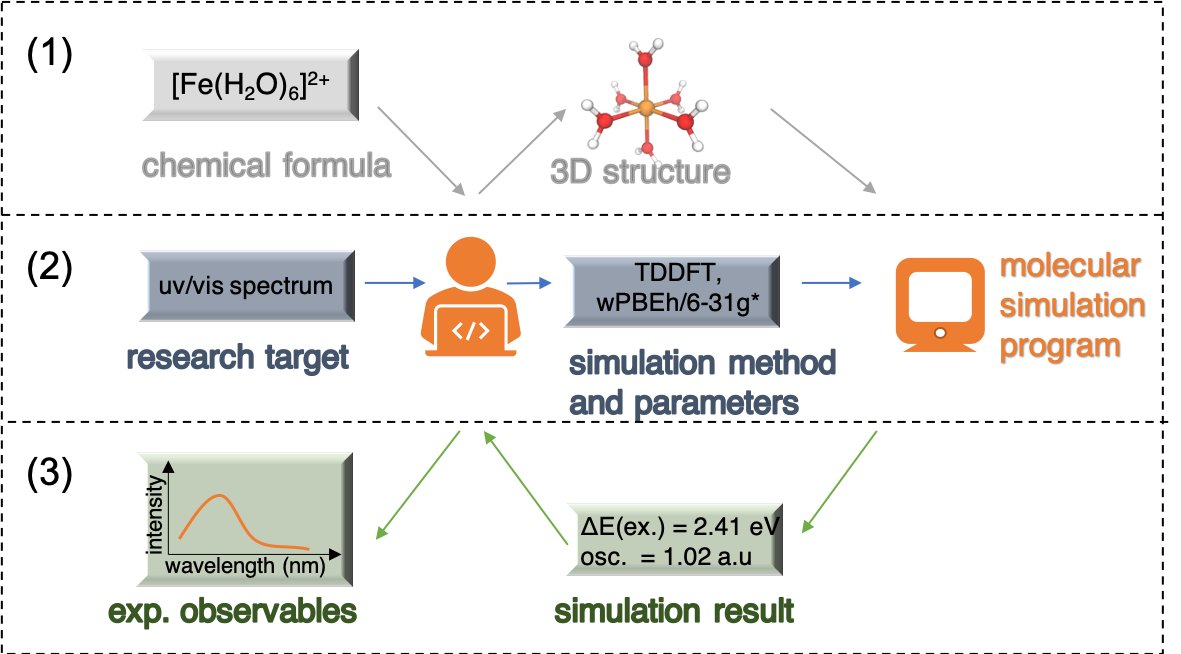Tutorial 0. Basics of running molecular simulation

Learning objectives

• Run a simple quantum chemistry calculation
• Understand the different aspects a researcher can contribute to a computational project.

Introduction

Instead of introducing the theories and models of different types of molecular simulation, we will start with an example to show a typical simulation task.A researcher gets involved in a quantum chemistry calculation task from multiple aspects:

1. Preparation of the representation of the molecule. We tell the molecular simulation program what system we study. Depending on the specific simulation program, the required representation of the molecule could be a 3D structure or some other type of information (e.g. chemical informatics formats, features for machine learning models).

2. Selection of simulation methods and parameters and prepare input files. Based on the research target, we make the plan about what type of simulation to run, what level of theory to apply, which programs to use, and which simulation parameters to set.

3. Analysis of simulation result. The output file generated by a simulation program is usually long and the useful information is hidden between lines. We need to read / post-process the output file to extract that information. Some text processing with scripts (Python/bash/perl etc.) is often useful.

And we develop theoretical methods and build the simulation packages! However, these tutorials will first focus on running simulations rather than methods development.

In future tutorials we will cover techniques for each of the three aspects.

Try and Learn

Here we will go over the above described process for one simple example: finding out the equilibrium structure (O-H bond length and H-O-H angle) of a water molecule.

(1) Preparation of the representation of the molecule.

Since our target is the equilibrium structure, we do need some 3D structure information. One simple and commonly used format is the .xyz format. It describes the element symbol and location (in cartesian coordinates) of each atom in the molecule.

Here we just guessed a structure of the water molecule (in unit Angstrom). Let’s save it as a file water.xyz

(2) Selection of simulation methods and parameters and prepare input files.

Density functional theory (DFT) has good balance between accuracy and efficiency for the description of ground state properties of small organic molecules. Here we run DFT geometry optimization with B3LYP functional and 6-31g* basis set, with an open source package: ORCA (Please download and install it on your computer).

Read the ORCA input library related to geometry optimization and DFT and learn to write an input file to run the calculation, or use the following input file:

Save the file as water_geoopt.in

Then run the calculation in the command line (assuming you have successfully installed ORCA and set the environment).

(3) Analysis of simulation result.

Read through the output file of your calculation and look for the optimized structure and the energy of the optimized structure. What are the O-H bond length and H-O-H angle?

Hints: Look for the line saying “OPTIMIZATION RUN DONE”, and then search backward for lines describing the structure.

Written on July 7, 2020